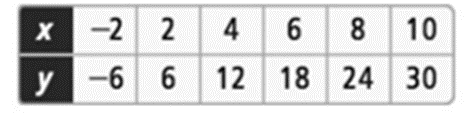Mathematics
Easy

Question

# Does the table of values represent an inverse variation?Hint:

## The correct answer is: No

### In contrast to direct variation, when changes in one quantity cause changes in another immediately, in inverse variation, the first quantity changes in the opposite direction of the other. Therefore, the relationship between the first and second quantities is inverse.If x and y are two variables, then according to the inverse variation:y = k/x      or      xy = kHere we have given the table which has certain values. In order to find inverse variation, lets multiply x and y, we get:.xy:-2 x -6 = 122 x 6 = 124 x 12 = 48So here we can see that the products are not constant. So, it is not an inverse variation.

Here we understood the concept of inverse variation with an example. It is basically the mathematical relationship between two variables that can be expressed by an equation in which the product of two variables is equal to a constant. So here in this table, we can see that the products are not constant. So, it is not an inverse variation.

### Related Questions to study#### With Turito Foundation.#### Get an Expert Advice From Turito.